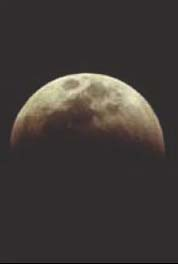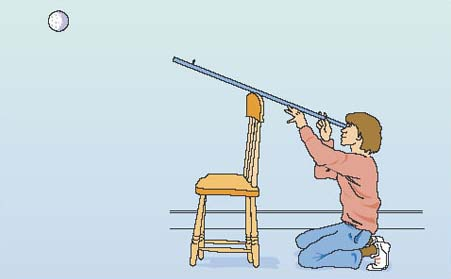The Sun

This free course is available to start right now. Review the full course description and key learning outcomes and create an account and enrol if you want a free statement of participation.

Free course

# 3.2 Angular size, actual size and distance

The angular size of an object is determined uniquely by its actual size and its distance from the observer. For an object of fixed size, the larger the distance, the smaller the angular size. For objects at a fixed distance, the larger the actual size of an object, the larger its angular size. For objects with small angular sizes, such as typical astronomical objects, the precise relationship between angular size, actual size and distance is well approximated by the equation:

angular size = (actual size ÷ distance)

However, when using this equation you must be very careful about the units in which quantities are measured. If the actual size and the distance are measured in the same units (metres or kilometres, or anything else as long as it is used for both quantities), the angular size that you calculate will be in measured units called radians. A radian is equal to a little more than 57° so, in order to obtain angular sizes in degrees, the following approximation can be used (as long as the angular size is not too great):

angular size = 57 × (actual size ÷ distance)

The next question asks you to apply this expression to Figure 20.

## Question 6

• Calculate 57 × (actual size ÷ distance) for each of the objects in Figure 20.

The values are 57 × (1 m ÷ 5.7 m), 57 × (2 m ÷ 11.4 m), 57 × (3 m ÷ 17.1 m), which is 10° in each case (as expected).

## Units, numbers and physical quantities

Much of astronomy concerns quantities such as temperatures, distances, diameters and angular sizes. In all of these cases, units of measurement are important. Physical quantities are generally the result of multiplying together a number and a unit of measurement. Thus a distance such as 5.2 kilometres is really the result of multiplying the number 5.2 by the unit of distance known as the kilometre. There are many units of measurement in common use, so, whenever you quote the value of a physical quantity, you should always take care to include the unit as well as the number multiplying that unit. It is no use being told that a distance is 5.2 if you don't know whether that means 5.2 centimetres or 5.2 kilometres. The unit is at least as important as the number.

In scientific work there are several internationally agreed conventions for the definition of units and the way in which units should be used and represented when writing down the values of physical quantities. The most widely adopted system of units is known as SI, which stands for Systeme International. This is based on seven carefully defined units that include the metre (for length), the second (for time) and the kilogram (for mass). The other four base units relate to luminous intensity (i.e. brightness), quantity of matter, electric current and temperature, the unit of which is the same size as the degree Celsius but starts from a different zero value and is called the kelvin.

The recognised abbreviations for the metre, the second and the kilogram are m, s and kg, respectively. In all cases, units should be written in the singular form, so it should really be 5.2 kilometre or 5.2 km, rather than 5.2 kilometres, and certainly never 5.2 kms, since that might be misinterpreted as 5.2 × 1 km × 1 s.

In calculations, units should be treated in the same way as numbers, so the result of dividing 6.0 km by 3.0 s is 2.0 km/s, which can be read as 2.0 kilometre per second.

Units, such as km/s, that result from combining the base units are called derived units. The most common derived units are sometimes given their own names and symbols. The angular unit known as a radian is an example, since angular size can be equated to the result of dividing one length (a diameter) by another length (a distance). The more familiar unit of angle - the degree - is said to be a supplementary unit since it is defined as a specific fraction (a little less than 1/57) of a radian.

To do the next activity, you need to know that the Moon's diameter is 3476 km. You may wonder how this can be measured from the Earth. In principle, it is a surprisingly easy measurement to make. First, you have to find the diameter of the Earth, which can be worked out by measuring how much its surface curves. You may be surprised to learn that this measurement was made in about 235 BC by the Greek astronomer Eratosthenes, and that his value was quite close to our modern measurement of 12756 km (for the equatorial diameter, which is slightly bigger than the polar diameter). The sizes of the Earth and the Moon can be compared by looking at the Earth's shadow on the Moon's surface during a partial eclipse of the Moon (see Figure 21). A careful measurement of this kind reveals that the Earth's diameter is 3.67 times that of the Moon.Figure 21 A partial eclipse of the Moon.

## Activity 4 The distance to the Moon

0 hours 30 minutes

This activity needs to be done when the Moon is clearly visible in the sky. (It need not be done at night and, in fact, can be easier in the day or at twilight.)

DO NOT ATTEMPT THIS ACTIVITY ON THE SUN.

You will need the following items:

• a selection of coins (e.g. 1p, 5p and 10p);

• a straight rod (e.g. a piece of dowelling or a garden cane) at least 2 m long;

• a tape measure at least 2 m long;

• a ruler marked in centimetres and millimetres;

• some Blu-Tack® or plasticine;

• a pocket calculator.

Set up an arrangement with a coin fixed to a rod so that the coin just 'eclipses' the Moon. Figure 22 shows one possible set-up.Figure 22 One possible arrangement for eclipsing the Moon.

Observing from one end of the rod, try different coins until you find one that is the right size to eclipse the Moon when fixed somewhere on the rod. Then adjust the position of the coin until it just blocks your view of the Moon. (This is less easy than it sounds, as there will always be some haze visible around the edge of the coin - try to get the best match.)

Measure the distance from the coin to the end of the rod where you have placed your eye, and measure the coin's diameter. Record your values like this:

Diameter of coin = .................... mm

Distance of coin = .................... mm

You now have the measurements that will enable you to calculate the angular size of a coin that has the same angular size as the Moon.

Use your two measurements on the coin to calculate its angular size in degrees, using the formula introduced earlier, adapted to the current case, i.e.:

angular size of coin = 57° × (diameter of coin ÷ distance of coin) = ....................

Your answer should be about half a degree (0.5°). Any value between 0.4° and 0.6° is fine. This is also your measurement of the angular size of the Moon. So, write down:

angular size of Moon in degrees = ....................

The next step is to calculate the distance to the Moon. Just as for the coin:

angular size of Moon = 57° × (diameter of coin ÷ distance of Moon) = ....................

This equation can be rearranged to give:

distance of Moon = 57° × (diameter of Moon ÷ angular size of Moon in degrees).

(Take this on trust if you cannot see it.) Now calculate the distance to the Moon, using your value for its angular size and 3476 km for its diameter.

Distance of Moon = 57° × (.................... ÷ ....................) = ....................km..

You might like to compare your result with the accurately measured value of the Moon's distance: 384 500 km. It is unlikely that you would have got very close to this answer, but even with such a quick and fairly rough measurement, your answer should show that the Moon's distance is a few hundred thousand km.

Here are some typical results from this activity:

• diameter of coin = 1.7 cm (UK 5 pence coin)

• distance of coin = 182 cm

• distance ÷ diameter = 107.058 82 on a calculator (near enough 107).

So, for something whose angular size is that of the Moon (half a degree):

• distance = 107 × diameter

• diameter of Moon = 3476 km.

Therefore, distance of Moon = 107 × 3476 km, i.e. distance of Moon = 371 932 km (near enough 372 000 km).

To eclipse the Moon, the distance of the coin needs to be roughly one hundred times its diameter. If you tried using a coin larger than 2 cm diameter, it would need to be more than 2 m from your eye and would not fit on a 2 m rod.

Our result is quite close to the accurately measured value of 384 500 km; yours may be closer, or not quite so close. Provided you got a value of a few hundred thousand kilometres, that is reasonable. If your value was very different, check back through your calculations to see whether you have made a mistake, and look again at your measurements.

The technique used in Activity 4 could also be used to work out the Sun's distance if you knew its size. However, under no circumstances should you try Activity 4 on the Sun because it would seriously damage your eyes.

S194_1